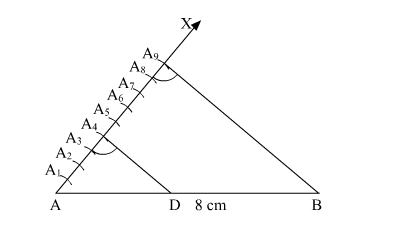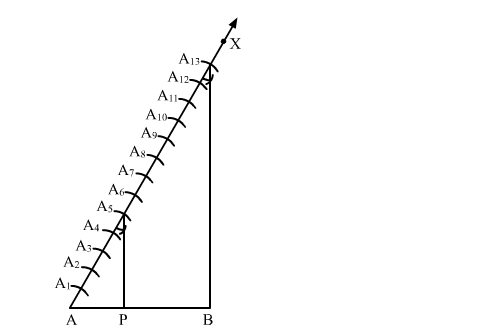# Draw a line segment of length 8 cm and divide it internally in the ratio 4 : 5.`
Question:

(i) Draw a line segment of length 8 cm and divide it internally in the ratio 4 : 5.

(ii) Draw a line segment of length 7.6 cm and divide it in the ratio 5 : 8. Measure the two parts.

Solution:

(i) Steps of Construction:

Step 1. Draw a line segment AB = 8 cm

Step 2. Draw a ray AX, making an acute angle ">BAX.

Step 3. Along AX, mark (4 + 5 =) 9 points A1, A2, A3, A4, A5, A6, A7, A8 and A9 such that

AA= A1A2 = A2A= A3A4 = A4A5 = A5A6 = A6A7 = A7A= A8A9

Step 4. Join A9B.

Step 5. From $A_{4}$, draw $A_{4} D$ parallel to $A_{9} B$ (draw an angle equal to $\angle A A_{9} B$ ), meeting $A B$ in $D$.Here, D is the point on AB which divides it in the ratio 4 : 5.

(ii) Steps of Construction:

Step 1. Draw a line segment AB = 7.6 cm

Step 2. Draw a ray $A X$, making an acute angle $\angle B A X$.

Step 3. Along AX, mark (5 + 8 =) 13 points A1, A2, A3, A4, A5, A6, A7, A8, A9, A10, A11, A12 and A13 such that

AA= A1A2 = A2A= A3A4 = A4A5 = A5A6 = A6A7 = A7A= A8A9 = A9A10 = A10A11 = A11A12 = A12A13

Step 4. Join A13B.

Step 5. From $A_{5}$, draw $A_{5} P$ parallel to $A_{13} B$ (draw an angle equal to $\angle A A_{13} B$ ), meeting $A B$ in $P$.Here, P is the point on AB which divides it in the ratio 5 : 8.

∴ Length of AP = 2.9 cm (Approx)

Length of BP = 4.7 cm (Approx)